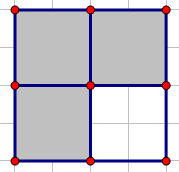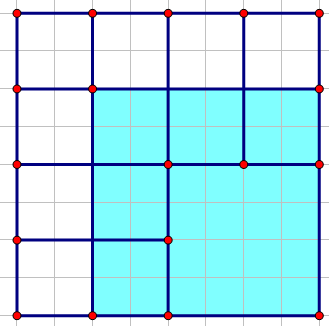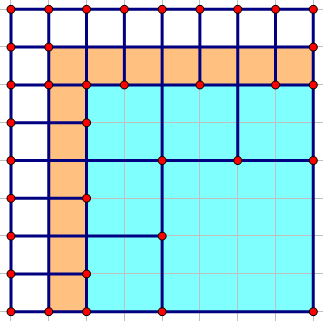Codeforces 传送门

## 『解析』

1. 先求一下最少次数
这种情况的最少操作次数为 $1+3+7+…+(2^i-1)$，感性理解一下——
当 $i=n-1$ 时显然最少需要进行 $1$ 次操作，这样就会变成：如果进一步让 $i=n-2$，那么我们就应该对上图的灰色块进行操作，也就是 $3$ 次。……以此类推，就会得到上面的式子~
2. 然后求最大次数
显然（一般来说）在最少次数的基础下我们还可以再进行一些不会对答案造成影响的操作——也就是对除了左下角到左上角再到右上角的路径上的正方形（最左边、最上边的正方形），我们最多可以把它们全部剪成$1 * 1$的~
令 $f(siz)$ 为将边长为 $2^{siz}$ 的正方形剪成 $1 * 1$ 的操作次数，那么我们发现 $2^{siz}$ 的边长剪 $1$ 次会变成 $4$ 个 $2^{siz-1}$，再剪 $4$ 次会变成 $16$ 个 $2^{siz-2}$……
以此类推，我们可以得到 $f(siz)=1+4+4^2+…+4^{siz-1}$ 。
假设现在正方形的边长为 $2^a$，左上角的正方形为 $2^b(b<a)$，如果按照最小方法剪，那么正方形可能长这样：上面的蓝色部分就是我们可以乱剪（不会对答案造成影响）的正方形，假设我们已经算出来了这一块蓝色部分全部剪成 $1 * 1$ 的操作次数，然后如果按照最小方法继续剪，它就会变成这样：上面的橙色部分就是相较上一次剪切多出来的可以任意操作而不会影响答案的正方形（假设它们的大小不是 $1 * 1$），那么我们要算这一次可以任意剪切的次数就可以根据上一次（蓝色部分）加上这次（橙色部分）全部剪成 $1 * 1$ 的操作次数~
而我们发现橙色正方形的边长就是我们的路径上的正方形边长，所以可以直接套用 $f()$ 函数计算。至于个数……假设上一次多出来的正方形（蓝色里面除去右下角的正方形）的个数为 $tmp’$ ，那么这次多出来的正方形个数就是 $tmp=(tmp-1) * 4+5$，找规律嘛~
那么最大操作数就是最少操作数加上将这些（橙色和蓝色）正方形剪成 $1 * 1$ 的正方形的操作数。